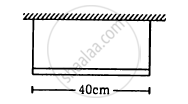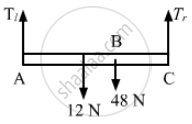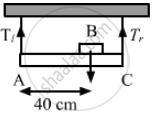Department of Pre-University Education, KarnatakaPUC Karnataka Science Class 11
Advertisement Remove all ads

# A Uniform Horizontal Rod of Length 40 Cm and Mass 1⋅2 Kg is Supported by Two Identical Wires as Shown in Figure (15-e9). Where Should a Mass of 4⋅8 Kg Be Placed on the - Physics

Sum

A uniform horizontal rod of length 40 cm and mass 1⋅2 kg is supported by two identical wires as shown in figure. Where should a mass of 4⋅8 kg be placed on the rod so that the same tuning fork may excite the wire on left into its fundamental vibrations and that on right into its first overtone? Take g = 10 m s−2.Advertisement Remove all ads

#### Solution

Given:
Length of the rod (L) = 40 cm = 0.40 m
Mass of the rod (m) = 1.2 kg
Let the mass of 4.8 kg be placed at x distance from the left.
As per the question, frequency on the left side = f0
Frequency on the right side = 2f0
Let tension be T1 and T2 on the left and the right side, respectively.

$\therefore \frac{1}{2L}\sqrt{\frac{T_1}{m}} = \frac{2}{2L}\sqrt{\frac{T_2}{m}}$

$\Rightarrow \sqrt{\frac{T_1}{T_2}} = 2$

$\Rightarrow \frac{T_1}{T_2} = 4 . . . (1)$
From the free body diagram:$T_1 + T_2 = 48 + 12 = 60 N$

$\Rightarrow 4 T_2 + T_2 = 5 T_2 = 60 N \left[ \text{ using equation } \left( 1 \right) \right]$

$\therefore T_2 = 12 N$

$\text{ and } T_1 = 48 N$
Now, taking moment about point A:$T_2 \times \left( 0 . 4 \right) = 48x + 12 \times 0 . 2$

$\Rightarrow 4 . 8 = 48x - 2 . 4$

$\Rightarrow 4 . 8x = 2 . 4$

$\Rightarrow x = \frac{2 . 4}{4 . 8} = \frac{1}{20} m = 5 \text{ cm }$
Therefore, the mass should be placed at a distance of 5 cm from the left end.

Concept: Wave Motion
Is there an error in this question or solution?
Advertisement Remove all ads

#### APPEARS IN

HC Verma Class 11, 12 Concepts of Physics 1
Chapter 15 Wave Motion and Waves on a String
Q 48 | Page 326
Advertisement Remove all ads
Advertisement Remove all ads
Share
Notifications

View all notifications

Forgot password?